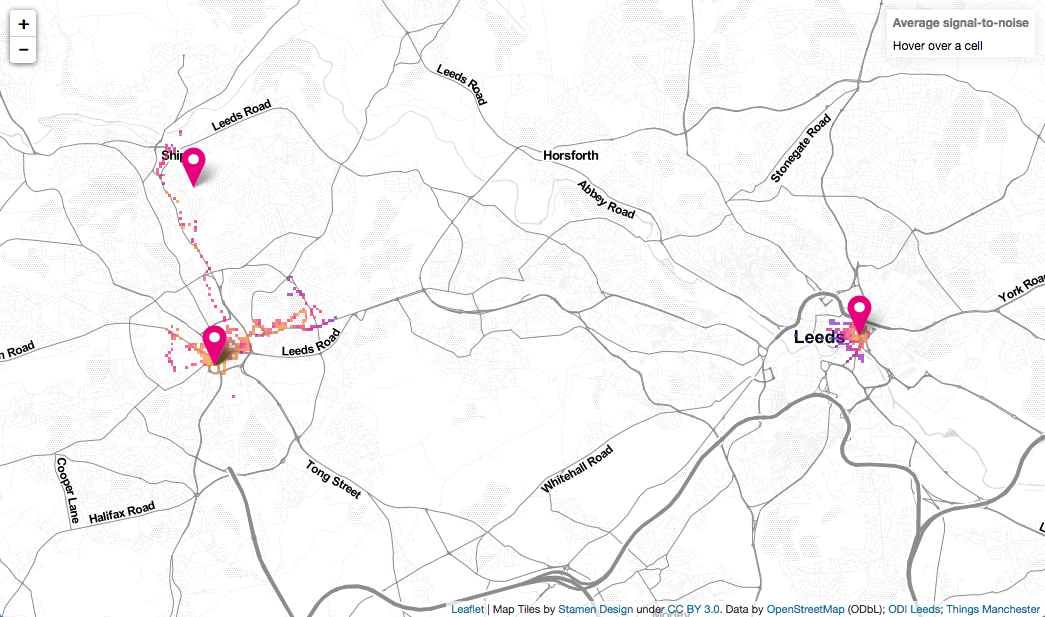# Things coverage map

When we started to build our flood network in Bradford, we needed to know where to place our sensors. The sensors needed to be on points in the river where we wanted to study, where there was access for installation, and where they could see a Things Network gateway. One gateway was installed on top of Margaret McMillan Tower in central Bradford and the second on a mast, up on the hill, at Wrose. Although the gateways, in theory, can communicate with a device over 10km away, Bradford is quite hilly. That meant it was likely that the topology would affect the range. We needed to know what the actual coverage was.

We bought an Adeunis field test device 868 which has a GPS and can be set up to transmit a packet every 30 seconds. The plan was to travel around Bradford sending packets with known locations and recording the signal strength recorded by the gateway(s) that received it. After setting up the keys for the device, we created a Node-RED instance to receive the packets and process them. It turns out that the instructions for the device didn't fully describe the binary/hex data blob from the device. After a bit of head scratching, I was able to decipher the full string of bytes. Here is the decoding function node I use in Node-RED:

``````msg.payload = JSON.parse(msg.payload);

function Hex2Bin(n){if(!checkHex(n))return 0;return parseInt(n,16).toString(2);}
function checkHex(n){return/^[0-9A-Fa-f]{1,64}\$/.test(n);}
function checkBin(n){return/^{1,64}\$/.test(n)}
function Bin2Dec(n){if(!checkBin(n))return 0;return parseInt(n,2);}
function Bin2Arr(str){ var a = new Array(str.length); for(var i = 0; i < str.length; i++){ a[i] = (str.substr(i,1) == "1" ? 1 : 0); } return a; }

var a = Hex2Bin(str);
var arr = Bin2Arr(a);

"temperature": arr,
"trigger_acc": arr,
"trigger_button": arr,
"gps": arr,
"up_ctr": arr,
"dn_ctr": arr,
"battery": arr
}
};

// Get temperature
var i = 8;

payload.ranger.T = (Bin2Dec(arr[i++]) ? -1 : 1)*Bin2Dec(a.substr(i,7));
i += 8;
}

// Get latitude
var lat_d = parseInt(str.substr(i/4,2));
i += 8;
var lat_m = parseInt(str.substr(i/4,2))
i += 8;
var lat_s = (parseInt(str.substr(i/4,3)));
i += 12;
var sign = (str.substr(1/4,1)=="1" ? -1 : 1);
i += 4;

// Get longitude
var lon_d = parseInt(str.substr(i/4,3));
i += 12;
var lon_m = parseInt(str.substr(i/4,2));
i += 8;
var lon_s = parseInt(str.substr(i/4,2));
i += 8;
if(typeof lon_s!=="number") lon_s = 0;
if(typeof lon_m!=="number") lon_m = 0;
if(typeof lon_d!=="number") lon_d = 0;
sign = (str.substr(i/4,1)=="1" ? -1 : 1);
i += 4;

}
i += 8;
}
i += 8;
}
i += 16;
}

}

msg.topic = "ODI Leeds Ranger";

return msg;
``````

I then pass this into a second function node that packages this up with the information about the gateways before storing the result.

``````var j = {'hw':msg.payload.hardware_serial,"ctr":msg.payload.counter,'time':msg.payload.metadata.time,"gps":msg.payload.ranger.gps,"T":msg.payload.ranger.T,"gateways":msg.payload.metadata.gateways};
// Truncate the latitude/longitude as we don't need the precision
msg.json = JSON.stringify(j).replace(/\"\;/,'"');
return msg;
``````

One thing to note is that the device only uses 6 bytes for longitude in the form DDMMXX where DD are the decimal degrees, MM are the decimal minutes and XX are hundredths of a minute of arc. That gives the position resolution as a 6000th of a degree which works out as about 12 metres at 53°N.

With a growing database of packets, I then needed a way to visualise them. Being from the Open Data Institute, I like to make use of open mapping so decided to make a heatmap using Leaflet.js and OpenStreetMap. The map code takes an array of [lat,lon,snr] values and puts them into square bins (correcting for the fact that the longitudes get squashed together at high latitudes). I then find the mean value for each bin, convert this to a colour, and construct a geojson object that gets drawn on the map.

Over the past few weeks we've done a lot of walking around Leeds and Bradford to start to get an idea of what the coverage looks like and how it differs between an externally mounted Kerlink gateway (Bradford) and an internal MultiTech gateway (Leeds). Initially we tried cycling but we were covering too much ground between packets being sent. Still, it is a good excuse to get out on foot.